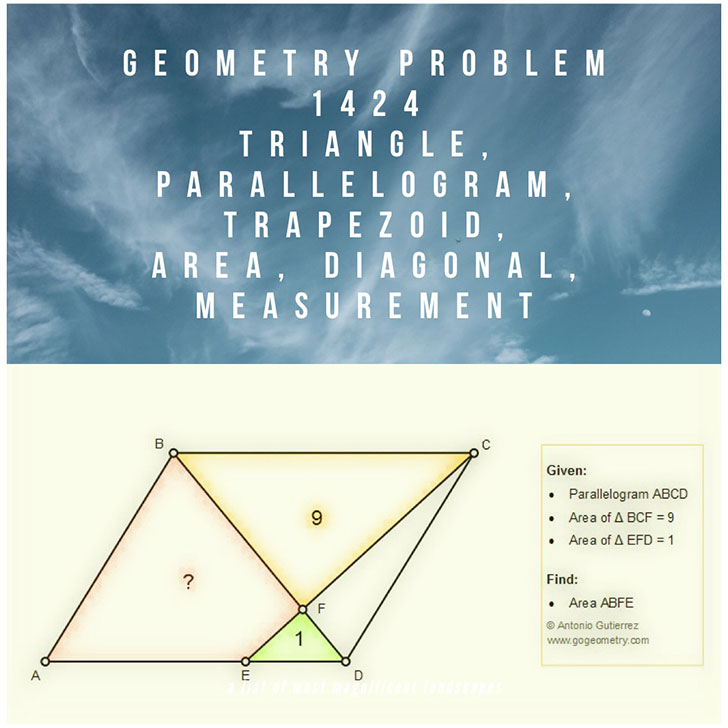# Geometry Problem 1424: Triangle, Parallelogram, Trapezoid, Area, Diagonal, Measurement

The figure below shows a parallelogram ABCD. E is a point on AD and BD intersects CE at F. If the area of triangle BCF is 9 and the area of triangle EFD is 1, find the area of quadrilateral ABFE.### Geometric Art of Problem 1424: Sketching, Typography, iPad Apps### Isolines or Contour Lines of Problem 1424An isoline or contour line of a function of two variables is a curve along which the function has a constant value. Isolines usually have a parallel trend.